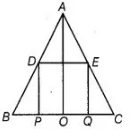# D and E are the mid-points of`
Question:

D and E are the mid-points of the sides AB and AC of ΔABC and 0 is any point on side BC. 0 is joined to A. If P and Q are the mid-points of OB and OC respectively, then DEQP is

(a) a square

(b) a rectangle

(c) a rhombus

(d) a parallelogram

Thinking Process

Use the mid-point theorem i.e., the line segment joining the mid-points of two sides of a triangle is parallel to the third side and is half of it.

Solution:

(d) In ΔABC, D and E are the mid-points of sides AB and AC, respectively.

By mid-point theorem,

DE || BC …(i)

DE = 1/2 BC

Then, $D E=\frac{1}{2}[B P+P O+O Q+Q C]$

$D E=\frac{1}{2}[2 P O+2 O Q]$

[since, $P$ and $Q$ are the mid-points of $O B$ and $O C$ respectively]

$\Rightarrow \quad D E=P O+O Q$

$\Rightarrow \quad D E=P Q$

Now, in $\triangle A O C, Q$ and $E$ are the mid-points of $O C$ and $A C$ respectively.

[by mid-point theorem]......(iii)Similarly, in $\triangle A B O, P D \| A O$ and $P D=\frac{1}{2} A O$

[by mid-point theorem].....(iv)

From Eqs. (iii) and (iv),

$E Q \| P D$ and $E Q=P D$

From Eqs. (i) and (ii), $D E \| B C$ (or $D E \| P Q$ ) and $D E=P Q$

Hence, $D E Q P$ is a parallelogram.